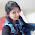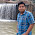## Thursday, April 02, 2015

### 3 years difference of Simple Interest and Compound Interest

In this video I have derived the formula of finding the difference between the simple interest and compound interest accumulated in the time period of three years. After deriving the formula the example of two questions has been shown in shown I have calculated the answer with both the conceptual method and correlating it with the formulae.

Question 1. Calculate the difference between the Simple Interest and Compound Interest for a time period of three years on a principle of Rs. 1000

Question 2. The difference between the Simple Interest and Compound Interest for 3 years on a sum of Rs. 1000.

1.Thank you for the videos.

2.sir in this video you had written formula of compound interest as:- P[1+R/100]^n-P but when you are calculating the difference between SI & CI there you had only written P[1+R/100]^n and you had not written -P alongwith that.

1.When we finding diff between ci and si 'p' got cancelled

Add a Comment or Query